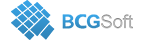BCGControlBar Pro for MFC
 virtual BOOL CBCGPChartTrendFormula::OnCalculateYValue ( double dblXVal, CBCGPChartValue & dblYVal )
inlineprotectedvirtual

Called to calculate Y value for a formula y = f(x).

Override this method in a derived class and return TRUE to implement a custom formula. dblYVal should be empty if an Y value is not defined for dblXVal (for example, y = log(x) can't be calculated for x <= 0).

Parameters
 dblXVal Specifies X value. dblYVal Contains resulting Y value when the method returns.